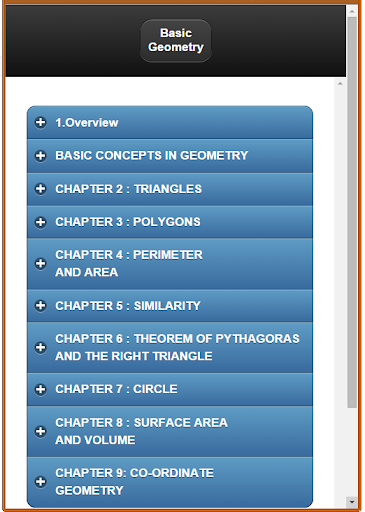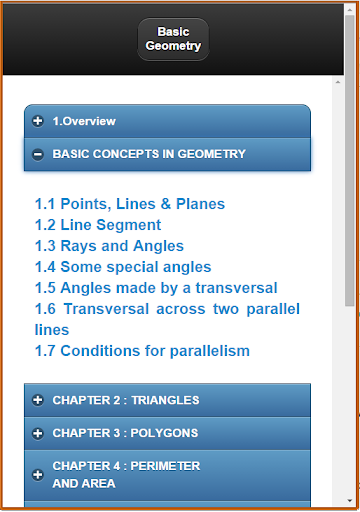1.1 Points, Lines & Planes
1.2 Line Segment
1.3 Rays and Angles
1.4 Some special angles
1.5 Angles made by a transversal
1.6 Transversal across two parallel lines
1.7 Conditions for parallelism
2.1 Introduction to Triangle
2.2 Sum of the angles of a triangle
2.3 Types of triangles
2.4 Altitude, Median and Angle Bisector
2.5 Congruence of triangles
2.6 Sides opposite congruent angles
3.1 Definition of polygon
3.2 Terminology
3.3 Sum of Interior angles of a Polygon
3.4 Sum of exterior angles of a Polygon
3.5 Trapezoids
3.6 Parallelograms
Conditions for a parallelogram
3.7 Square, Rectangle and Rhombus
4.1 Perimeter
4.2 Square
4.3 Rectangle
4.4 Parallelogram
4.5 Triangle
4.6 Trapezoids
4.7 Circles
5.1 Introduction to similarity
5.2 Ratio and Proportionality
5.3 Similar Polygons
5.4 Basic Proportionality Theorem
5.5 Angle bisector theorem
5.6 Similar Triangles
5.7 Properties of Similar triangles
6.1 The Right Triangle
6.2 The Theorem of Pythagoras
6.3 Special Right Triangles
7.1 Introduction to circle
7.2 Lines of a Circle
7.3 Arcs
7.4 Inscribed angles
7.5 Some properties of tangents, secants and chords
7.6 Chords and their arcs
7.7 Segments of chords secants and tangents
7.8 Lengths of arcs and areas of sectors
8.1 Introduction to Solid Geometry
8.2 Prism
8.3 The cuboid and the cube
8.4 Circular Cylinders
8.5 Pyramids
8.6 Right circular cone
8.7 Sphere
9.1 Points and Co-ordinates
9.2 Co-ordinates and Axes